Next: The Chaotic Pendulum Up: Lunar Motion Previous: Perturbed Lunar Motion

# Description of Lunar Motion

In order to better understand the perturbed lunar motion derived in the previous section, it is helpful to introduce the concept of the mean moon. This is an imaginary body which orbits the Earth, in the ecliptic plane, at a steady angular velocity that is equal to the Moon's mean orbital angular velocity,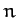. Likewise, the mean sun is a second imaginary body which orbits the Earth, in the ecliptic plane, at a steady angular velocity that is equal to the Sun's mean orbital angular velocity,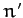. Thus, the ecliptic longitudes of the mean moon and the mean sun are(1216)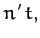(1217)

respectively. Here, for the sake of simplicity, and also for the sake of consistency with our previous analysis, we have assumed that both objects are located at ecliptic longitude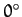at time.

Now, from Equation (1213), to first-order in small parameters, the lunar perigee corresponds to, whereis an integer. However, this condition can also be written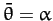, where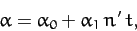(1218)

and, making use of Equation (1207), together with the definition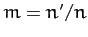,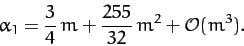(1219)

Thus, we can identify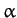as the mean ecliptic longitude of the perigee. Moreover, according to Equation (1218), the perigee precesses (i.e., its longitude increases in time) at the mean rate ofdegrees per year. (Of course, a year corresponds to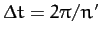.) Furthermore, it is clear that this precession is entirely due to the perturbing influence of the Sun, since it only depends on the parameter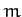, which is a measure of this influence. Given that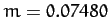, we find that the perigee advances bydegrees per year. Hence, we predict that the perigee completes a full circuit about the Earth everyyears. In fact, the lunar perigee completes a full circuit every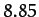years. Our prediction is somewhat inaccurate because our previous analysis neglected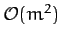, and smaller, contributions to the parameter[see Equation (1207)], and these turn out to be significant.

From Equation (1215), to first-order in small parameters, the Moon passes through its ascending node when, whereis an integer. However, this condition can also be written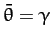, where(1220)

and, making use of Equation (1209),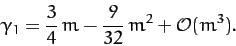(1221)

Thus, we can identify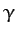as the mean ecliptic longitude of the ascending node. Moreover, according to Equation (1220), the ascending node regresses (i.e., its longitude decreases in time) at the mean rate ofdegrees per year. As before, it is clear that this regression is entirely due to the perturbing influence of the Sun. Moreover, we find that the ascending node retreats by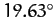degrees per year. Hence, we predict that the ascending node completes a full circuit about the Earth everyyears. In fact, the lunar ascending node completes a full circuit everyyears, so our prediction is fairly accurate.

It is helpful to introduce the lunar mean anomaly,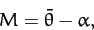(1222)

which is defined as the angular distance (in longitude) between the mean Moon and the perigee. It is also helpful to introduce the lunar mean argument of latitude,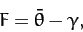(1223)

which is defined as the angular distance (in longitude) between the mean Moon and the ascending node. Finally, it is helpful to introduce the mean elongation of the Moon,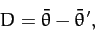(1224)

which is defined as the difference between the longitudes of the mean Moon and the mean Sun.

When expressed in terms of,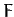, and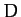, our previous expression (1214) for the true ecliptic longitude of the Moon becomes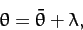(1225)

where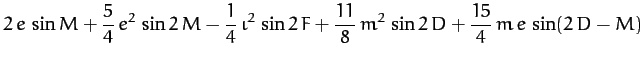(1226)

is the angular distance (in longitude) between the Moon and the mean Moon. The first three terms on the right-hand side of the above expression are Keplerian (i.e., they are independent of the perturbing action of the Sun). In fact, the first is due to the eccentricity of the lunar orbit (i.e., the fact that the geometric center of the orbit is slightly shifted from the Earth), the second is due to the ellipticity of the orbit (i.e., the fact that the orbit is slightly non-circular), and the third is due to the slight inclination of the orbit to the ecliptic plane. However, the final two terms are caused by the perturbing action of the Sun. In fact, the fourth term corresponds to variation, whilst the fifth corresponds to evection. Note that variation attains its maximal amplitude around the so-called octant points, at which the Moon's disk is either one-quarter or three-quarters illuminated (i.e., when,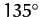,, or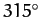). Conversely, the amplitude of variation is zero around the so-called quadrant points, at which the Moon's disk is either fully illuminated, half illuminated, or not illuminated at all (i.e., when,,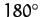, or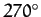). Evection can be thought of as causing a slight reduction in the eccentricity of the lunar orbit around the times of the new moon and the full moon (i.e.,and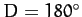), and causing a corresponding slight increase in the eccentricity around the times of the first and last quarter moons (i.e.,and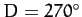). This follows because the evection term in Equation (1226) augments the eccentricity term,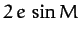, when, and reduces the term when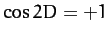. The variation and evection terms appearing in expression (1226) oscillate sinusoidally with periods of half a synodic month,or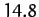days, anddays, respectively. These periods are in good agreement with observations. Finally, the amplitudes of the variation and evection terms (calculated usingand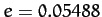) areand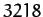arc seconds, respectively. However, the observed amplitudes are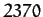and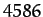arc seconds, respectively. It turns out that our expressions for these amplitudes are somewhat inaccurate because, for the sake of simplicity, we have only calculated the lowest order (in) contributions to them. Recall that we also neglected the slight eccentricity,, of the Sun's apparent orbit about the Earth in our calculation. In fact, the eccentricity of the solar orbit gives rise to a small addition termon the right-hand side of (1226), where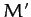is the Sun's mean anomaly. This term, which is known as the annual equation, oscillates with a period of a solar year, and has an amplitude of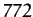arc seconds.

When expressed in terms ofandour previous expression (1215) for the ecliptic latitude of the Moon becomes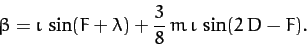(1227)

The first term on the right-hand side of this expression is Keplerian (i.e., it is independent of the perturbing influence of the Sun). However, the final term, which is known as evection in latitude, is due to the Sun's action. Evection in latitude can be thought of as causing a slight increase in the inclination of the lunar orbit to the ecliptic at the times of the first and last quarter moons, and a slight decrease at the times of the new moon and the full moon. The evection in latitude term oscillates sinusoidally with a period of 32.3 days, and has an amplitude of 521 arc seconds. This period is in good agreement with observations. However, the observed amplitude of the evection in latitude term is 624 arc seconds. As before, our expression for the amplitude is somewhat inaccurate because we have only calculated the lowest order (in) contribution.Next: The Chaotic Pendulum Up: Lunar Motion Previous: Perturbed Lunar Motion
Richard Fitzpatrick 2011-03-31